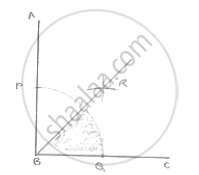# Using Protractor, Draw a Right Angle. Bisect It to Get an Angle of Measure 45°. - Mathematics

Using protractor, draw a right angle. Bisect it to get an angle of measure 45°.

#### SolutionSteps of construction:
1. Draw an angle ABC of 90°
2. With center B and any radius, draw an arc which intersects AB at P and BC at Q
3. With center P and Q and radius more than 1/2PQ draw two arcs, which intersect each other at R.
4. Join RB

∴ ∠RBC = 45°

Concept: Basic Constructions
Is there an error in this question or solution?

#### APPEARS IN

RD Sharma Mathematics for Class 9
Chapter 16 Constructions
Exercise 16.2 | Q 4 | Page 9

Share